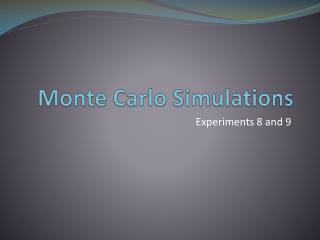DownloadDownload PresentationMonte Carlo Simulations

# Monte Carlo Simulations

Télécharger la présentation## Monte Carlo Simulations

- - - - - - - - - - - - - - - - - - - - - - - - - - - E N D - - - - - - - - - - - - - - - - - - - - - - - - - - -
##### Presentation Transcript

1. Monte Carlo Simulations Experiments 8 and 9

2. Monte Carlo Simulation • Monte Carlo simulations in PSpice can be run as either: • a worst case analysis where the maximum deviation from the nominal values of each component are used in the calculations • a statistically-driven variation from the nominal values of the components using a bell shaped curve for the distribution of deviations.

3. Prior to Monte Carlo • Set up a Simulation Run using Analysis Setup • You must select one of the other types of analysis first • Click on DC Sweep • Pick either of the voltage sources to sweep • Make both the start and end • values equal to the nominal • value of the voltage source • Pick any value for the • increment. • Click OK.

4. Analysis Setup • Select Monte Carlo/Worst Case • In the pop-up window, enter the number of runs in MC Run • At least 500 is recommended for statically reasons • Not more than 2000 due to run time issues Do not unselect the Bias Point Detail

5. Monte Carlo or Worst Case • In Output Var: box, enter V(node name) or V(component:node) • This is the advantage of labeling the nodes. So, you will enter V(X) and V(Y) for the two Monte Carlo simulations that are required. • Note that you will also have to put a voltage marker on to the circuit schematic no matter what you put as the output variable • If you have selected a DC Sweep, select DC as the Analysis Type.

6. Monte Carlo or Worst Case • Select All on the MC Options. • Then, click OK. • Once the schematic is saved, you are ready to run the simulation.

7. Open the Plot Window Click OK.

8. Output Plot is a Histogram

9. Histogram • The shape of the histogram and the values of the mean, maximum, and minimum voltages at node X will differ every time you run the simulation because a random number generator is used to determine the values of each of the five resistors in every one of the runs. • The differences in the mean, maximum, and minimum values are negligible if sufficient number of runs are entered in the MC Run box when you set up the simulation. • The shape of the histogram becomes more symmetrical as the number of runs approaches 2000.

10. Your Experimental Results • If your voltage supplies are exactly +5 V and +9 V, then the voltages that you measure at nodes X and Y should fall somewhere within the range from the minimum to maximum values of the voltages calculated during the Monte Carlo simulation. • If you measure the actual values of the +5 V and +9 V supplies and enter them into the simulation, then there is a 100% likelihood that your measurements will fall within this range.